Get instant live expert help with Excel or Google Sheets“My Excelchat expert helped me in less than 20 minutes, saving me what would have been 5 hours of work!”

#### Post your problem and you’ll get expert help in seconds.

Your message must be at least 40 characters
Our professional experts are available now. Your privacy is guaranteed.

# Learn How to Use the DAYS Function in ExcelRead time: 15 minutes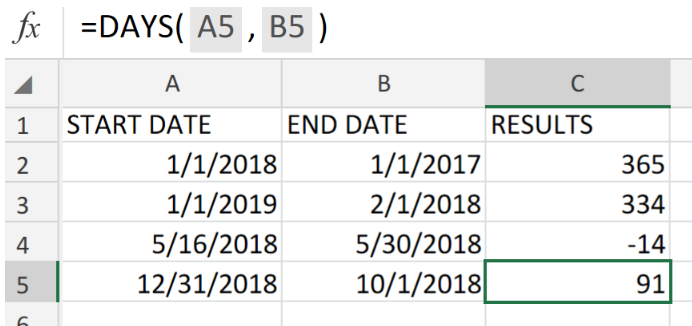Figure 1. Excel Days Function.

If we want to obtain a number representing days occurring between two specific dates in Excel, we must make use of the DAYS function.

## Formula Syntax

`=DAYS (end_date, start_date)`

• start_date = A specified date from which Excel begins to count.
• end_date = A specified date where Excel is to stop it’s count.

Determine the total number of days occurring between two dates by using the Excel DAYS function.

## How to use the Excel DAYS Function.

Follow the 3 simple steps described below to understand the application of the DAYS Function;

1. In our worksheet example below, our purpose is to determine the total number of days between the dates in the START DATE and END DATE columns.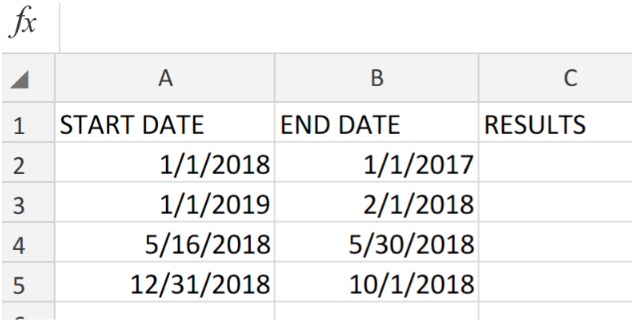Figure 2. Excel Days Function.

Ensure that you have provided Excel with empty cells to return our desired results.

1. The DAYS formula we will type in cell C2 of our worksheet, is;

`=DAYS(A2,B2)`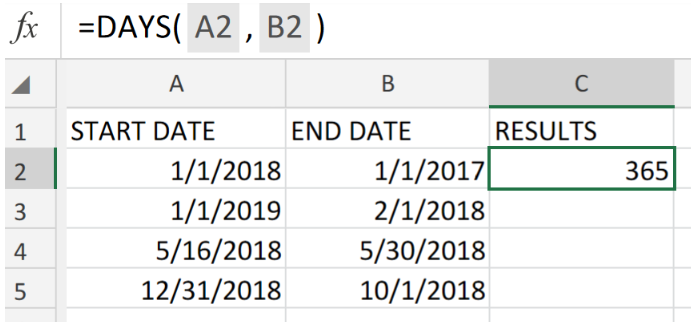Figure 3. Excel Days Function.

The Excel Days formula calculated the total number of days occurring between the dates in cells A2:B2, as 365.

1. Copy a  modified version of the Days formula we used above, down the other cells in the RESULTS column to get the other results.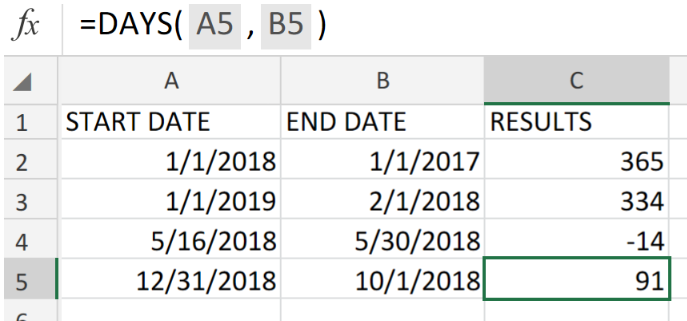Figure 4. Excel Days Function.

Even if we define the start and end dates as text in our Days formula syntax, DAYS function will still calculate the number of days between those two dates.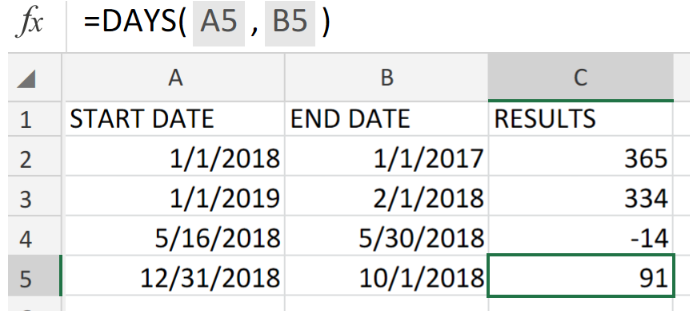Figure 5. Final Result.

## Instant Connection to an Expert through our Excelchat Service:

Our live Excelchat Service is here for you. We have Excel Experts available 24/7 to answer any Excel questions you may have. Guaranteed connection within 30 seconds and a customized solution for you within 20 minutes.

### Did this post not answer your question? Get a solution from connecting with the expert.Another blog reader asked this question today on Excelchat:
Related blogs
Solution examplesI have multiple columns on my spread sheet and want to switch from A-Z to Z-A based on values in column A
Solved by V. H. in 38 minsConcat formula is incorrectly formatting the date in column C. Currently column B has dd/mm/yyyy and I require mm/dd/yyyy
Solved by Z. Y. in 31 minsI need a formula that will allow me to calc before a certain date and after a certain date. =if(A2<=DATE(2015,8,27),(F2*1.14),(F2*1.15)) It just multiplies column F with 1.15. I'm assuming I need to bring in the date column into the equation?
Solved by M. Q. in 20 minsI need assistance setting up a time sheet formula where I can create a column of contiguous dates down the left hand side of the spread sheet. So I can copy a contiguous column of dates, rather than entering each date manually. I would only need to have work days in the list, ie Monday to Friday inc. with a couple of spare lines between each week.
Solved by Z. Y. in 40 minsExample: If a loan closes between October 1st & 15th, the first mortgage payment is due November 1st. If it closes between October 16th & 31st, the first payment is due December 1st.
Solved by S. S. in 15 mins## Subscribe to Excelchat.coAnother blog reader asked this question today on Excelchat: